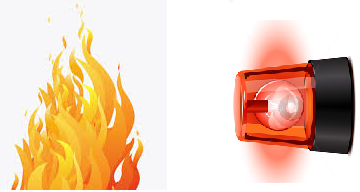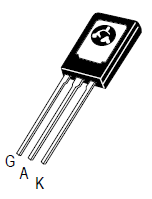﻿ How to Build a Heat Alarm Circuit ﻿# How to Build a Heat Alarm CircuitIn this project, we will go over how to build a heat alarm circuit.

This is a circuit that will go off once the heat that the circuit is exposed to reaches a certain level. Once heat increases beyond a certain level, a loud buzzer will go off, alerting us to this.

In doing this, this circuit will alert us to when it is exposed to a dangerous level of heat. Once alerted to this, the user can take action such as cutting off the heat source, if possible, or shutting off the circuit.

So the alarm will not go off when the circuit is exposed to regular room temperature but if a high heat source were to come upon it, it will go off if the heat is pretty significant.

So a heat alarm has good real-world application.

Components Needed

• 100KΩ Thermistor
• C106B SCR
• LED
• 3 AA Batteries

What we will use for this circuit is a 100KΩ thermistor. This can be obtained at taydaelectronics at the following link: Taydaelectronics- 100KΩ Thermistor.

A thermistor is a resistor whose resistance changes according to the amount of heat that it is exposed to. There are 2 types of thermistors, NTC and PTC thermistors. NTC thermistors are thermistors in which the greater the amount of heat the thermistor is exposed to, the lower the resistance drops. So heat and resistance have an indirect relationship. The resistance falls as the heat increases. This is the type of thermistor we will use in our circuit.

The thermistor is the biggest part for the heat aspect of this circuit. It serves as a heat sensor. When the circuit is just exposed to room temperature and no significant source of heat, the thermistor offers a large amount of resistance. In fact, it offers the resistance that the thermistor is rated for. In our case, we are using a 100KΩ thermistor, so when the thermistor isn't exposed to any significant source of heat, it will offer a resistance near this rated value of 100KΩ. As the heat which the thermistor is exposed to increases, its resistance will begin to drop.

When at room temperature, since the resistance is so high, practically no current will flow through the circuit, so nothing turns on. If the circuit is exposed to more heat, then the resistance drops and then sufficient current is able to flow which allows electronic components to turn on.

With the thermistor focusing as the heat sensor, the other significant component is the silicon-controlled rectifier (SCR).

The SCR is a 3-pin device composed of a gate, an anode, and a cathode.Like a transistor, when the SCR receives sufficient voltage at its gate terminal, it conducts across from anode to cathode. Without this voltage at its gate, no current can flow from anode to cathode. With this gate voltage, current can flow and power the load connected to the anode of the SCR. However, unlike a transistor, a SCR is different in that once it receives sufficient voltage at its gate, it conducts indefinitely from anode to cathode- unless power is disconnected from the anode. So while a transistor needs ongoing current at its base or gate terminal to conduct current across its junction, an SCR only needs to be triggered once to remain on. Thus, it acts like a latching circuit in that once it is turned on, it latches on and stays on.

Looking at the above SCR, with it turned to its back surface, the pin to the leftmost is the gate terminal, the middle pin is the anode, and the rightmost pin is its cathode.

This is the datasheet for the SCR: C106B SCR Datasheet.

The thermistor will be connected to the gate of the SCR. When the heat increases to a certain level, the resistance drops, allowing current to flow through and trigger the gate of the SCR. The SCR, then triggered, will then turn on the load connected to it, which in our case is a buzzer or an LED.

### Heat Alarm Circuit Schematic

The schematic for the heat alarm circuit is shown below:This is the circuit we will build.

At room temperature, the thermistor will offer very high resistance, near its rated value of 100KΩ. At this resistance, practically no current flows so the SCR gate does not get triggered.

However, when the thermistor is exposed to a significant amount of heat, its resistance drops significantly. Enough current now flows through the circuit to trigger the gate of the SCR. Once the gate of the SCR is triggered, current now flows across from its anode to cathode and turns on the LED. Once triggered, the SCR stays on, just like an alarm, stimulating an alarm circuit.

And this is how this heat alarm circuit works.

Different variations can be done for this circuit. If you don't want an LED to go off but instead want an a buzzer to sound, swap out the LED with a buzzer. With a buzzer, you do not need a current-limiting resistor, but you may need more voltage, so you may have to modify the power. You can add any device that you want to go off once the SCR is triggered. Customize the circuit to suit your preferences.

We have built this actual circuit. To see how this circuit operates and its output in real life, see the video below:

In this circuit, if too much heat is applied, the LED turns on as an indicator that there is too much heat.

Related Resources